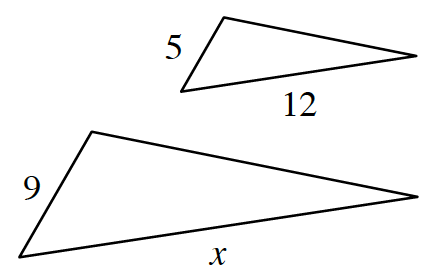### Home > GB8I > Chapter 9 Unit 3 > Lesson CC3: 9.2.1 > Problem9-65

9-65.

Eric set up this ratio for two similar triangles:

$\frac { x } { 12 } = \frac { 5 } { 9 }$

He solved the problem and found $x ≈ 6.67$. What was his mistake? Homework Help ✎

Examine the ratios Eric created. Did he set up his ratios correctly?